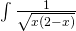## Integration fun with MATLAB and Mathematica

September 13th, 2011 | Categories: general math, just for fun, mathematica, matlab | Tags:

Consider this indefinite integralFeed it to MATLAB’s symbolic toolbox:

int(1/sqrt(x*(2 - x)))

ans =
asin(x - 1)


Feed it to Mathematica 8.0.1:

Integrate[1/Sqrt[x (2 - x)], x] // InputForm

(2*Sqrt[-2 + x]*Sqrt[x]*Log[Sqrt[-2 + x] + Sqrt[x]])/Sqrt[-((-2 + x)*x)]


Let x=1.2 in both results:

MATLAB's answer evaluates to 0.2014
Mathematica's answer evaluates to -1.36944 + 0.693147 I


Discuss!

1. …and Sagemath gives -arcsin(-x + 1)… Numerically gives the same (0.201357920790331). Could it be related with a kind of default number field for variables (Real or Complex)?

2. What happens if you assume x is real in Mathematica?

3. eh :What happens if you assume x is real in Mathematica?

(and in the range (0,2))

4. eh :What happens if you assume x is real in Mathematica?

This is interesting:

http://www.wolframalpha.com/input/?i=Integrate%5B1%2FSqrt%5Bx+%282+-+x%29%5D%2C+x%5D

5. The integrand has a pole at x = 2. Perhaps Mathematica and Matlab has chosen different convention in dealing with the pole.

The real parts of the two results differs by a constant function and the imaginary parts by a step function:
http://goo.gl/H1vew

6. Maple gives 0.2014, too. But, this is a limit value(x->1.2), not the exact(x=1.2).
Still not sure if Mathematica gives the exact value, is it? Interesting post, thank u.

> a := int(1/sqrt(x*(2-x)), x);
arcsin(-1 + x)
> ans := evalf(limit(a, x = 1.2), 50);
0.20135792079033079145512555221762341024003808140223 #50 digits

7. In:= Simplify[Integrate[1/Sqrt[x (2 – x)], x]] // InputForm

Assuming x is in range (0,2) makes no difference:

In:= Simplify[Integrate[1/Sqrt[x (2 – x)], x]] // InputForm

Out//InputForm=
(2*Sqrt[-2 + x]*Sqrt[x]*Log[Sqrt[-2 + x] + Sqrt[x]])/Sqrt[-((-2 + x)*x)]

In:= Simplify[%, 0 <= x <= 2] // InputForm

Out//InputForm=
(2*I)*Log[Sqrt[-2 + x] + Sqrt[x]]

8. @expfunc
Rafa has already said the numerical answer. I read it just now, sorry.

9. Putting the integration on Wolfram Alpha (http://www.wolframalpha.com/input/?i=Integrate%5B1%2FSqrt%5Bx+%282+-+x%29%5D%2C+x%5D) and clicking on “Show Steps” it indicates that both expressions (symbolic results of Mathematica and MATLAB) are equivalent – for restricted values, whatever it is :-)

10. Mathematica result = Matlab result + (-pi/2 + i * ln(2) sign(2 – x)) for x in Reals.

11. Mathematica result = Matlab result + (-pi/2 + i * ln(2) sign(2 – x)) for complex x.

12. It really seems to be the same up to the constant: http://www.wolframalpha.com/input/?i=plot+{asin%28x+-+1%29%2C%5B%282+Sqrt%5B-2+%2B+x%5D+Sqrt%5Bx%5D+Log%5BSqrt%5B-2+%2B+x%5D+%2B+Sqrt%5Bx%5D%5D%29%2F+++Sqrt%5B-%28%28-2+%2B+x%29+x%29%5D%5D}&cdf=1

13. Filling 1.2 into the integral does not make any sense; you need both bounds to get the ‘area’. You can add any number to the integral of an equation.

14. The two solutions differ by a complex constant (something like 2i*log(2) + Pi/2). That’s fine.

So, the integral evaluated between 1.9 and 1.2 is approximately .9184115942083033952315515036.

That is
1.119769514998634186686677056 – 0.2013579207903307914551255522 [first way], or
(-0.4510268117962624325446446358 + 0.6931471805599453094172321215 i) –
( -1.369438406004565827776196139 + 0.6931471805599453094172321214 i) [second way]

15. @Sander Huisman
Making x=1.2 makes sense into the solution of the indefinite integral, assuming that it has an analytic solution.

16. Disagree; it does not make any sense to fill in 1 value; the integral itself does not say anything. It’s like asking for the area at a point. You need two point…

17. @Sander Huisman
I meant set x=1.2 to the solution (asin(x-1)) not to the integral (it’s indefinite).

18. @Rafa
That is what I meant; substituting a value in the solution is like asking for the area at a point; does not make any sense.

19. It seems that the answer is incorrent in principle. If one take the ArcSin function in logarithm form then the results differ form it by i*ln(i) + i*ln(2). It is strange.

It is also interesting to note that to calculate arcsin in Mathematica by logarithm one should take not the principal value of the square root but one of the branche:

asin[z_]:=-i Log[ i ( Sqrt[Abs[z^2 -1]] Exp[i Arg[z^2-1]/2 + i Pi] +z ) ]

Probably this can shed the light to the problem.

20. @DataComputist
The integrand has branching point at x=2 (and x=0) not pole.

21. I think Sander has hit the nail on the head. Anti-differentiaion is up to a complex-constant, so in that sense, evaluating the antiderivative at a point is meaningless since you can add an arbitrary constant to the result and get whatever you like. Also since you can express 1 = sin(x)^2 + cos(x)^2, you can add a whole variety of “constant” trig functions, which can often make anti-derivatives look very different.

For instance, the derivative of 2 ArcSin[Sqrt[x]/Sqrt] is also 1/Sqrt[(2 – x) x]. This function evaluated at x=1.2 is ~ 1.77215.

All these functions are anti-derivatives locally.

It’s interesting to note that the Im parts of both functions are constant on (0,2) and the Re parts are constant elsewhere. So we should expect the integral to be strictly real on [0,2] and strictly imaginary on any interval not containing [0,2].

22. Tom :you can add a whole variety of “constant” trig functions, which can often make anti-derivatives look very different.

But Mathematica takes one (“special”) form of the constant from this “whole variety”.

23. So they give identical answers!
-1.36944 + 0.693147 I+Carb1 = 0.2014+Carb2.

The reason Mathematica expands the trigonomic function I think is simply because we consider for example
sqrt(3)/2 to be a better answer for a calculation then sin(2/3 pi). With the matlab answer you are “stuck” at the trigonomic functions, where with the Mathematica answer, you suddenly have the roots and logs, which could be considered a “better” answer to the question.

24. Very Interesting ! …..

PS : I reserve my right for particular comments on this one ! … LOL !